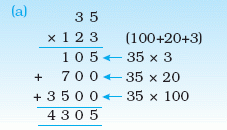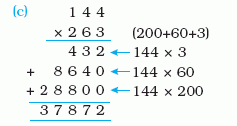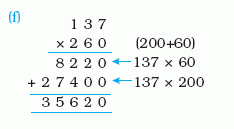Name: ___________________Date:___________________

 Email us to get an instant 20% discount on highly effective K-12 Math & English kwizNET Programs!

MEAP Preparation - Grade 6 Mathematics2.62 Multiplication - Three Digits

 Multiplying 2 digit and 3 digit numbersMultiplying 3 digit and 2 digit numbersMultiplying 3 digit and 3 digit numbersDirections: Multiply the numbers shown in the question. Use a separate sheet to show your work and write the answer in the box.Name: ___________________Date:___________________

MEAP Preparation - Grade 6 Mathematics2.62 Multiplication - Three Digits

 Question 1: 710 * 617

 Question 2: 714 * 988

 Question 3: 219 * 100

 Question 4: 248 * 71

 Question 5: 850 * 543

Question 6: This question is available to subscribers only!

Question 7: This question is available to subscribers only!

Question 8: This question is available to subscribers only!

Question 9: This question is available to subscribers only!

Question 10: This question is available to subscribers only!